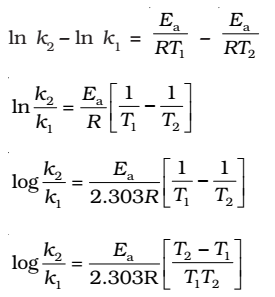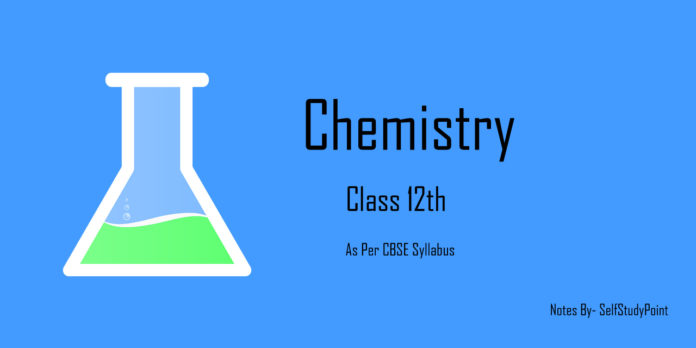# Arrhenius equation

Quantitively, the temperature dependence of the rate of a chemical reaction can be explained by Arrhenius equation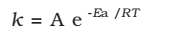where A is the Arrhenius factor or the frequency factor or pre-exponential factor. R is gas constant and Ea is activation energy measured in joules/mole.

The factor e-Ea/RT corresponds to the fraction of molecules that have the kinetic energy greater than Ea.

Thus, it has been found from Arrhenius equation that increasing the temperature or decreasing the activation energy will result in an increase in the rate of the reaction and an exponential increase in the rate constant.

Taking natural logarithm of both sides of equation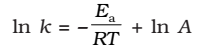The plot of ln k vs 1/T gives a straight line with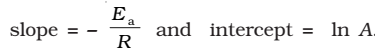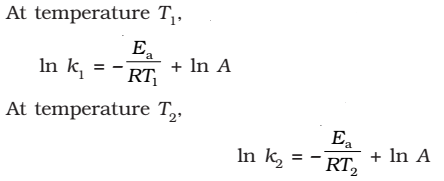(since A is constant for a given reaction)

k1and k2 are the values of rate constants at temperatures T1 and T2 respectively.

Subtracting equation form, we obtain